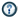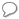xNewton's laws of motionOverview

Newton's laws of motion are three physical law
Physical law
A physical law or scientific law is "a theoretical principle deduced from particular facts, applicable to a defined group or class of phenomena, and expressible by the statement that a particular phenomenon always occurs if certain conditions be present." Physical laws are typically conclusions...

s that form the basis for classical mechanics
Classical mechanics
In physics, classical mechanics is one of the two major sub-fields of mechanics, which is concerned with the set of physical laws describing the motion of bodies under the action of a system of forces...

. They describe the relationship between the force
Force
In physics, a force is any influence that causes an object to undergo a change in speed, a change in direction, or a change in shape. In other words, a force is that which can cause an object with mass to change its velocity , i.e., to accelerate, or which can cause a flexible object to deform...

s acting on a body and its motion
Motion (physics)
In physics, motion is a change in position of an object with respect to time. Change in action is the result of an unbalanced force. Motion is typically described in terms of velocity, acceleration, displacement and time . An object's velocity cannot change unless it is acted upon by a force, as...

due to those forces. They have been expressed in several different ways over nearly three centuries, and can be summarized as follows:
1. First law: The velocity
Velocity
In physics, velocity is speed in a given direction. Speed describes only how fast an object is moving, whereas velocity gives both the speed and direction of the object's motion. To have a constant velocity, an object must have a constant speed and motion in a constant direction. Constant ...

of a body remains constant unless the body is acted upon by an external force.
2. Second law: The acceleration
Acceleration
In physics, acceleration is the rate of change of velocity with time. In one dimension, acceleration is the rate at which something speeds up or slows down. However, since velocity is a vector, acceleration describes the rate of change of both the magnitude and the direction of velocity. ...

a of a body is parallel
Parallel
-Mathematics and science:* Parallel , an imaginary east-west line circling a globe* Parallel circuits, as opposed to series* Parallel * Parallel evolution* Parallel transport* Parallel of declination, used in astronomy-Computing:...

and directly proportional to the net force
Force
In physics, a force is any influence that causes an object to undergo a change in speed, a change in direction, or a change in shape. In other words, a force is that which can cause an object with mass to change its velocity , i.e., to accelerate, or which can cause a flexible object to deform...

F and inversely proportional to the mass
Mass
Mass can be defined as a quantitive measure of the resistance an object has to change in its velocity.In physics, mass commonly refers to any of the following three properties of matter, which have been shown experimentally to be equivalent:...

m, i.e., F = ma.
3. Third law: The mutual forces of action and reaction between two bodies are equal, opposite and collinear.

The three laws of motion were first compiled by Sir Isaac Newton
Isaac Newton
Sir Isaac Newton PRS was an English physicist, mathematician, astronomer, natural philosopher, alchemist, and theologian, who has been "considered by many to be the greatest and most influential scientist who ever lived."...

in his work Philosophiæ Naturalis Principia Mathematica, first published on July 5, 1687.how does newtons laws of motion deal with the spport of basketball?DiscussionsWhat other phenomenonWere these laws published or were just passed down?Does NewtonWhat other laws did Newton come up?How many laws form Newton